Courses

# Concepts in Probability Mathematics Notes | EduRev

## Mathematics : Concepts in Probability Mathematics Notes | EduRev

The document Concepts in Probability Mathematics Notes | EduRev is a part of the Mathematics Course Additional Topics for IIT JAM Mathematics.
All you need of Mathematics at this link: Mathematics

Further Concepts in Probability
The study of probability mostly deals with combining different events and studying these events alongside each other. How these different events relate to each other determines the methods and rules to follow when we're studying their probabilities.
Events can be pided into two major categories dependent or Independent events.

Independent Events
When two events are said to be independent of each other, what this means is that the probability that one event occurs in no way affects the probability of the other event occurring. An example of two independent events is as follows; say you rolled a die and flipped a coin. The probability of getting any number face on the die in no way influences the probability of getting a head or a tail on the coin.

Dependent Events
When two events are said to be dependent, the probability of one event occurring influences the likelihood of the other event.

For example, if you were to draw a two cards from a deck of 52 cards. If on your first draw you had an ace and you put that aside, the probability of drawing an ace on the second draw is greatly changed because you drew an ace the first time. Let's calculate these different probabilities to see what's going on.
There are 4 Aces in a deck of 52 cards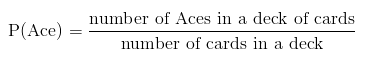On your first draw, the probability of getting an ace is given by: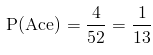If we don't return this card into the deck, the probability of drawing an ace on the second pick is given by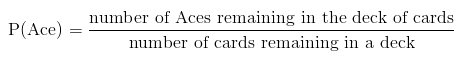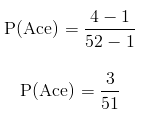As you can clearly see, the above two probabilities are different, so we say that the two events are dependent. The likelihood of the second event depends on what happens in the first event.

Conditional Probability
We have already defined dependent and independent events and seen how probability of one event relates to the probability of the other event.
Having those concepts in mind, we can now look at conditional probability.
Conditional probability deals with further defining dependence of events by looking at probability of an event given that some other event first occurs.

Conditional probability is denoted by the following:
P(B|A)
The above is read as the probability that B occurs given that A has already occurred.
The above is mathematically defined as: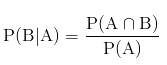Set Theory in Probability
A sample space is defined as a universal set of all possible outcomes from a given experiment.
Given two events A and B and given that these events are part of a sample space S. This sample space is represented as a set as in the diagram below.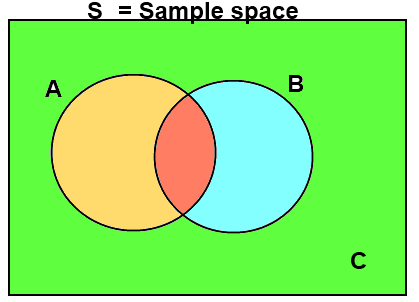The entire sample space of S is given by:
S = {A, B, C}
Remember the following from set theory: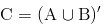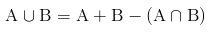The different regions of the set S can be explained as using the rules of probability.

Rules of Probability
When dealing with more than one event, there are certain rules that we must follow when studying probability of these events. These rules depend greatly on whether the events we are looking at are Independent or dependent on each other.
First acknowledge that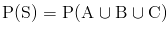Multiplication Rule (A∩B)
This region is referred to as 'A intersection B' and in probability; this region refers to the event that both A and B happen. When we use the word and we are referring to multiplication, thus A and B can be thought of as AxB or (using dot notation which is more popular in probability) A•B

If A and B are dependent events, the probability of this event happening can be calculated as shown below: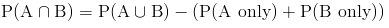If A and B are independent events, the probability of this event happening can be calculated as shown below: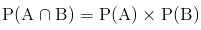Conditional probability for two independent events can be redefined using the relationship above to become: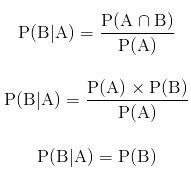The above is consistent with the definition of independent events, the occurrence of event A in no way influences the occurrence of event B, and so the probability that event B occurs given that event A has occurred is the same as the probability of event B.

In probability we refer to the addition operator (+) as or. Thus when we want to we want to define some event such that the event can be A or B, to find the probability of that event: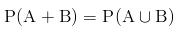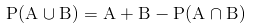Thus it follows that: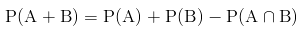But remember from set theory that and from the way we defined our sample space above: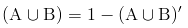and that: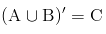So we can now redefine out event as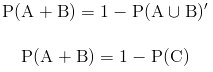The above is sometimes referred to as the subtraction rule.

Mutual Exclusivity
Certain special pairs of events have a unique relationship referred to as mutual exclusivity.
Two events are said to be mutually exclusive if they can't occur at the same time. For a given sample space, its either one or the other but not both. As a consequence, mutually exclusive events have their probability defined as follows: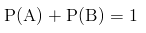An example of mutually exclusive events are the outcomes of a fair coin flip. When you flip a fair coin, you either get a head or a tail but not both, we can prove that these events are mutually exclusive by adding their probabilities: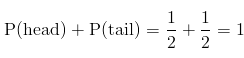For any given pair of events, if the sum of their probabilities is equal to one, then those two events are mutually exclusive.

Rules of Probability for Mutually Exclusive Events

• Multiplication Rule
From the definition of mutually exclusive events, we should quickly conclude the following: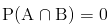As we defined above, the addition rule applies to mutually exclusive events as follows:
P(A + B) = 1
• Subtraction Rule
From the addition rule above, we can conclude that the subtraction rule for mutually exclusive events takes the form;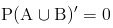Conditional Probability for Mutually Exclusive Events
We have defined conditional probability with the following equation: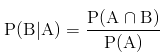We can redefine the above using the multiplication rule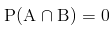hence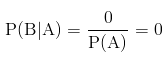Below is a venn diagram of a set containing two mutually exclusive events A and B.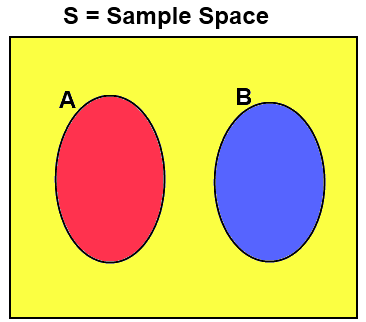Offer running on EduRev: Apply code STAYHOME200 to get INR 200 off on our premium plan EduRev Infinity!

40 docs

,

,

,

,

,

,

,

,

,

,

,

,

,

,

,

,

,

,

,

,

,

;